# Class 10 RD Sharma Solutions – Chapter 3 Pair of Linear Equations in Two Variables – Exercise 3.2 | Set 3

### (i) 2x + 3y = 12 and x – y = 1

Solution:

Given that, 2x + 3y = 12 and x – y = 1

Now, 2x + 3y = 12

x = (12-3y)/2

When y = 2, we get x = 3

When y = 4, we get x = 0

So, the following table giving points on the line 2x + 3y = 12

Now, x – y = 1

x = y + 1

When y = 0, we get x = 1

When y = 1, we get x = 2

So, the following table giving points on the line x – y = 1

So, the graph of the equations 2x + 3y = 12 and x – y = 1: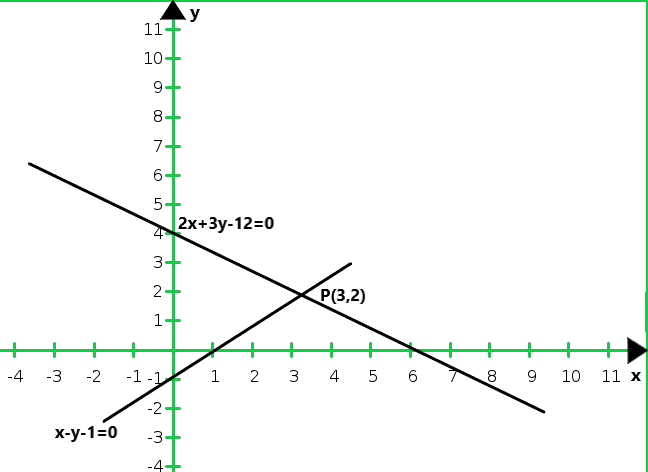From the graph we conclude that the two lines intersect at P (3, 2)

Hence, x = 3 and y = 2 is the solution of the given system of equations.

### (ii) 3x + 2y – 4 = 0 and 2x – 3y – 7 = 0

Solution:

Given that, 3x + 2y – 4 = 0 and 2x – 3y – 7 = 0

Now, 3x + 2y – 4 = 0

x = (4 – 2y)/3

When y = 5, we get x = – 2

When y = 8, we get x = – 4

So, the following table giving points on the line 3x + 2y – 4 = 0

We have,

2x – 3y – 7 = 0

x = (3y + 7)/2

When y = 1, we get x = 5

When y = -1, we get x = 2

So, the following table giving points on the line 2x – 3y – 7 = 0

So, the graph of the equations 3x + 2y – 4 = 0 and 2x – 3y – 7 = 0: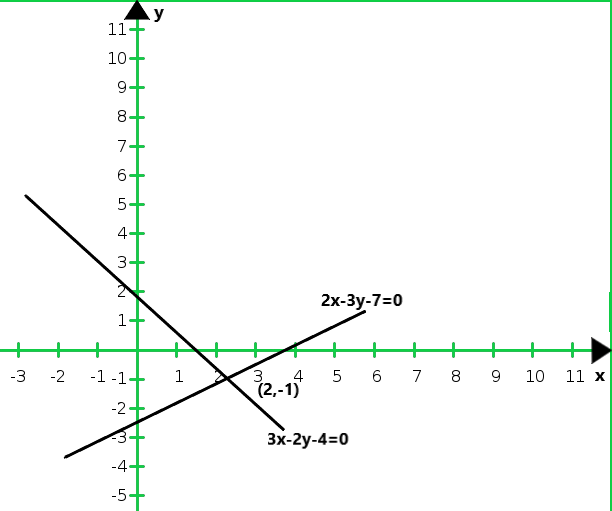From the graph we conclude that the two lines intersect at P(2,-1)

Hence, x = 2 and y = -1 is the solution of the given system of equations.

### (iii) 3x + 2y – 11 = 0 and 2x – 3y + 10 = 0

Solution:

Given that, 3x + 2y – 11 = 0 and 2x – 3y + 10 = 0

Now, 3x + 2y – 11 = 0

x = (11 – 2y)/3

When y = 1, we get x = – 3

When y = 4, we get x = 1

So, the following table giving points on the line 3x + 2y – 11 = 0

Now,

2x – 3y + 10 = 0

x = (3y-10)/2

When y = 0, we get x = – 5

When y = 2, we get x = – 2

So, the following table giving points on the line 2x – 3y + 10 = 0

So, the graph of the equations 3x + 2y – 11 = 0 and 2x – 3y + 10 = 0: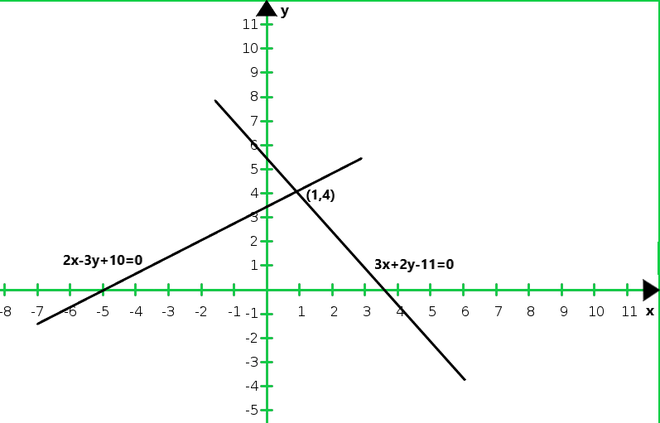From the graph we conclude that the two lines intersect at P(1, 4)

Hence, x = 1 and y = 4 is the solution of the given system of equations.

### 2x + 3y = 12 and x – y = 1

Solution:

Given that, 2x + 3y = 12 and x – y = 1

Now, 2x + 3y = 12

x = (12 – 3y)/2

When y = 0, we get x = 6

When y = 2, we get x = 3

So, the following table giving points on the line 2x + 3y = 12:

Now,

x – y = 1

x = 1 + y

When y = 0, we get x = 1

When y = -1, we get x = 0

So, the following table giving points on the line x – y = 1:

So, the graph of the equations 2x + 3y = 12 and x – y = 1: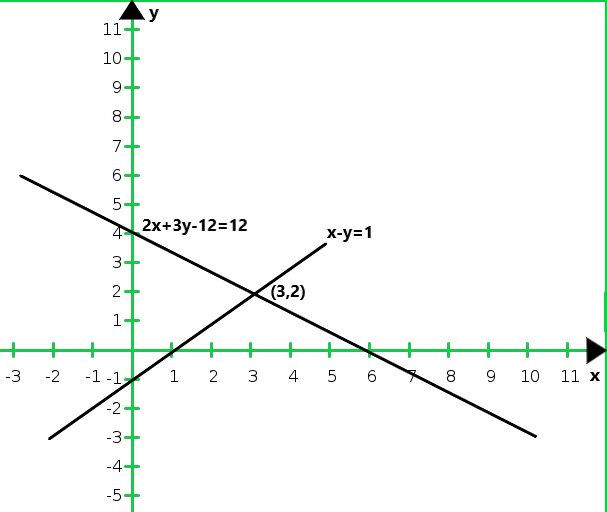From the graph we conclude that the two lines intersect at A (3, 2)

Also, we observe that the lines meet y-axis B (0, – 1) and C (0, 4)

Hence, the vertices of the required triangle are A (3, 2), B (0,-1), and C (0, 4).

### Question 25. Draw the graphs of x – y + 1 = 0 and 3x + 2y – 12 = 0. Determine the coordinates of the vertices of the triangle formed by these lines and x-axis and shade the triangular area. Calculate the area bounded by these lines and the x-axis.

Solution:

Given that, x – y + 1 = 0 and 3x + 2y – 12 = 0

Now, x – y + 1 = 0

x = y – 1

When y = 3, we get x = 2

When y = -1, we get x = -2

So, the following table giving points on the line x – y + 1 = 0

We have,

3x + 2y – 12 = 0

x = (12 – 2y)/3

When y = 6, we get x = 0

When y = 3, we get x = 2

So, the following table giving points on the line 3x + 2y – 12 = 0

So, the graph of the equations x – y + 1 = 0 and 3x + 2y – 12 = 0: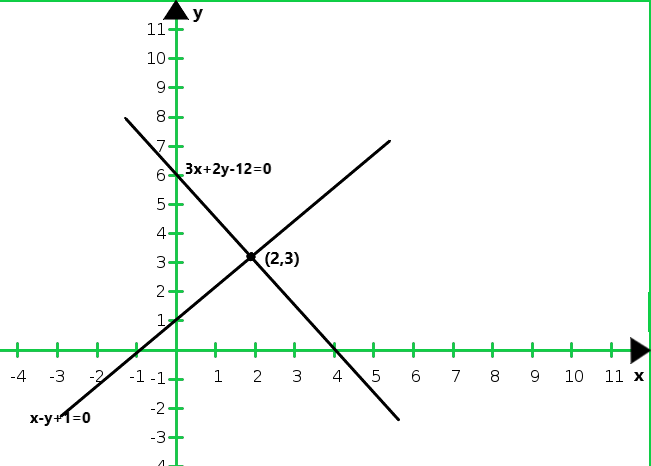From the graph we conclude that the two lines intersect at A(2, 3)

Also, we observe that the lines meet x-axis B(-1, 0) and C(4, 0)

So, x = 2 and y = 3 is the solution of the given system of equations.

AD is drawn perpendicular A on x-axis.

So,

AD = y-coordinate point A (2, 3)

AD = 3 and BC = 4- ( – 1) = 4 + 1 = 5

Hence, the area of the shaded region = 1/2 × base × altitude

1/2 × 5 × 3 = 15/2 = 7.5 sq. units

### Find the area bounded by these lines and x-axis.

Solution:

Given that, 4x – 3y + 4 = 0 and 4x + 3y – 20 = 0

Now, 4x – 3y + 4 = 0

x = (3y – 4)/4

When y = 0, we get x = -1

When y = 4, we get x = 2

So, the following table giving points on the line 4x – 3y + 4 = 0

Now,

4x + 3y – 20 = 0

x = (20 – 3y)/4

When y = 0, we get x = 5

When y = 4, we get x = 2

So, the following table giving points on the line 4x + 3y – 20 = 0

So, the graph of the equations 4x – 3y + 4 = 0 and 4x + 3y – 20 = 0: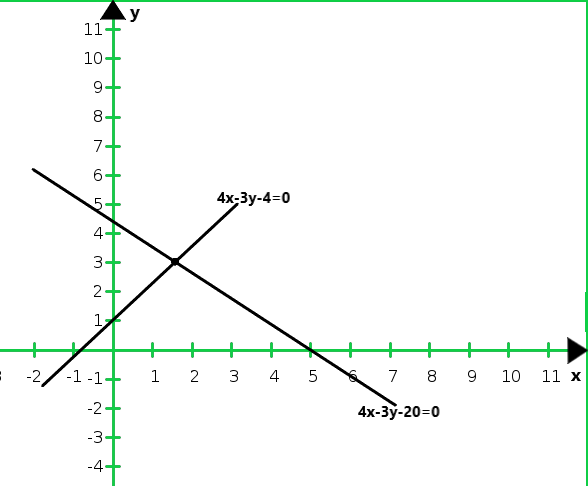From the graph we conclude that the two lines intersect at A (2, 4)

Also, we observe that the lines meet x-axis B (-1, 0) and C(5, 0)

So, x = 2 and y = 4 is the solution of the given system of equations.

AD is drawn perpendicular A on x-axis.

So, we get

AD = y-coordinate point A (2, 4)

AD = 3 and BC = 5 – (-1) = 4 + 1 = 6

Hence, the area of the shaded region =1/2 × base × altitude

1/2 × 6 × 4 = 12 sq. units

### Shade the region bounded by these lines and the y-axis. Also, find the area of the region bounded by these lines and the y-axis.

Solution:

Given that, 3x + y – 11 = 0 and x – y – 1 = 0

Now, 3x + y – 11 = 0

y = 11 – 3x

When x = 0, we get y = 11

When x = 3, we get y = 2

So, the following table giving points on the line 3x + y – 11 = 0

We have

x – y – 1 = 0

y = x – 1

When x = 0, we get y = -1

When x = 3, we get y = 2

So, the following table giving points on the line x – y – 1 = 0

So, the graph of the equations 3x + y – 11 = 0 and x – y – 1 = 0: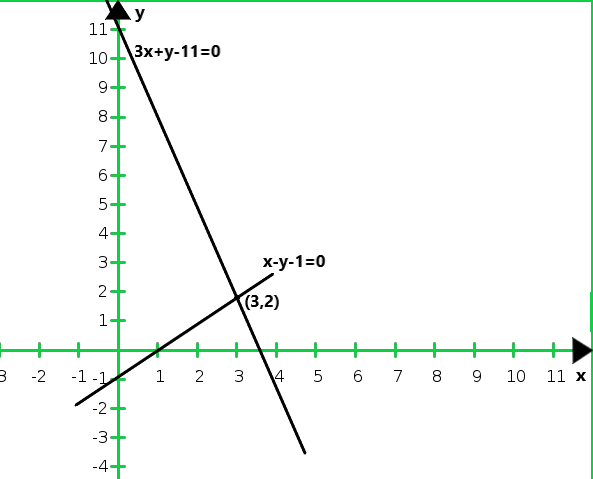From the graph we conclude that, the two lines intersect at A (3, 2)

We also observe that the lines meet y-axis B(0, 11) and C (0, – 1)

So, x = 3 and y = 2 is the solution of the given system of equations.

AD is drawn perpendicular A on x-axis. Clearly we have,

AD = y-coordinate point A(2, 4)

AD = 3 and BC = 11- (- 1) = 11 + 1 = 12

Hence, the area of the shaded region = 1/2 × base × altitude

1/2 × 12 × 3 = 18 sq. units

### Find the vertices of the triangle so obtained. Also, find the area of the triangle.

Solution:

Given that, 2x – 3y + 6 = 0, 2x + 3y – 18 = 0, and y – 2 = 0

Now, 2x – 3y + 6 = 0

x = (3y – 6)/2

When y = 0, we get x = -3

When y = 2, we get x = 0

So, the following table giving points on the line  2x – 3y + 6 = 0

Now,

2x + 3y – 18 = 0

x = 18-3y / 2

When y = 2, we get x = 6

When y = 6, we get x = 0

So, the following table giving points on the line 2x + 3y – 18 = 0

Now,

y – 2 = 0

y = -2

So, the graph of the equations 2x – 3y + 6 = 0, 2x + 3y – 18 = 0, and y – 2 = 0: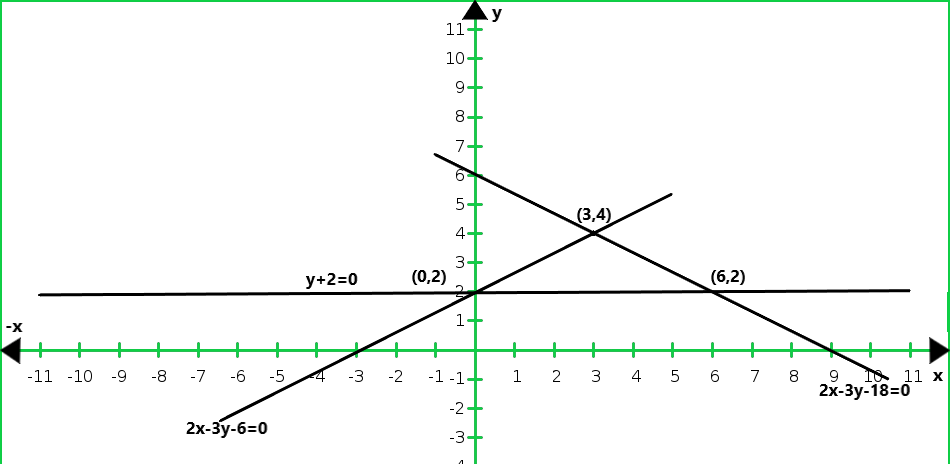From the graph of three equations, we conclude that the three lines taken in pairs

intersect each other at points A(3, 4), B(0, 2), and C(6, 2)

Hence, the vertices of the required triangle are (3, 4), (0, 2) and (6, 2)

From the graph, we have

AD = 4 – 2 = 2

BC = 6 – 0 = 6

Hence, the area of the shaded region = 1/2 × base × altitude

1/2 × 6 × 2 = 6 sq. units

### Also, find the area of the region bounded by these lines and y-axis.

Solution:

Given that, 2x – 3y + 6 = 0 and 2x + 3y – 18 = 0

Now, 2x – 3y + 6 = 0

y = (2x + 6)/3

When x = 0, we get y = 2

When x = -3, we get y = 0

So, the following table giving points on the line 2x – 3y + 6 = 0

Now,

2x + 3y – 18 = 0

x = (18 – 3y)/2

When y = 2, we get x = 6

When y = 6, we get x = 0

So, the following table giving points on the line 2x + 3y – 18 = 0

So, the graph of the equations 2x – 3y + 6 = 0 and 2x + 3y – 18 = 0: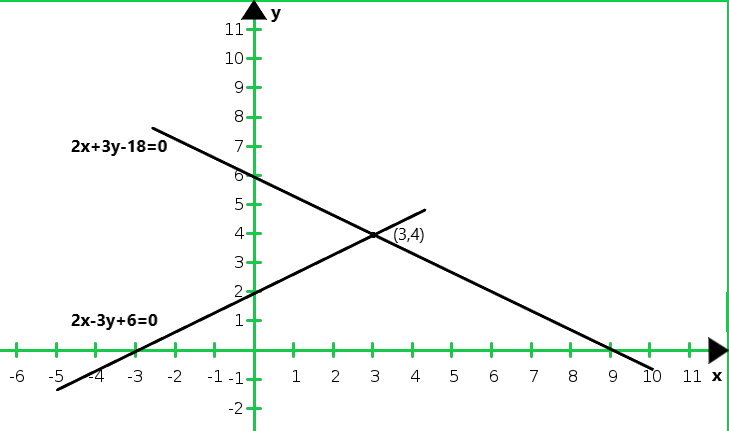From the graph we conclude that, the two lines intersect at A (3, 4).

Hence x = 3 and y = 4 is the solution of the given system of equations.

Also, from the graph, we have

AD = x-coordinate point A(3, 4) = 3

BC = 6 – 2 = 4

Hence, the area of the shaded region = 1/2 × base × altitude

1/2 × 4 × 3 = 6 sq. units

### Determine the vertices of triangle formed by the lines representing the above equation and the y-axis.

Solution:

Given that, 4x – 5y – 20 = 0 and 3x + 5y – 15 = 0

Now, 4x – 5y – 20 = 0

x = (5y + 20)/4

When y = 0, we get x = 5

When y = – 4, we get x = 0

So, the following table giving points on the line 4x – 5y – 20 = 0

We have

3x + 5y – 15 = 0

x = (5y + 20)/5

When y = 0, we get x = 5

When y = – 4, we get x = 0

So, the following table giving points on the line 3x + 5y – 15 = 0

So, the graph of the equations 4x – 5y – 20 = 0 and 3x + 5y – 15 = 0: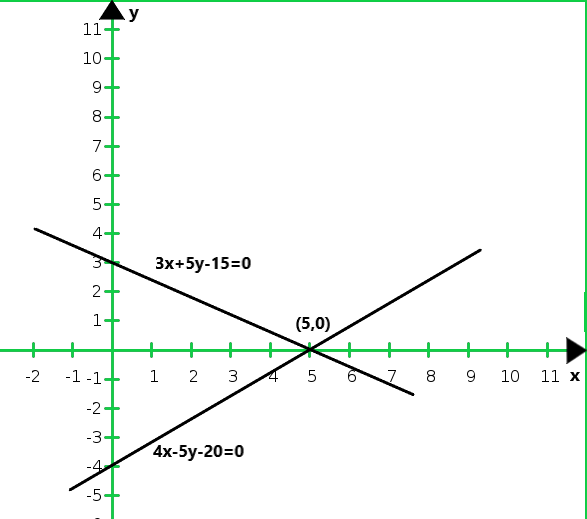From the graph we conclude that the two lines intersect at A(5, 0).

Hence, x – 5, y – 0 is the solution of the given system of equations.

So, the lines meet the y-axis at B(0, -4) and C(0, 3) respectively.

Hence, the vertices of the triangle are (5, 0), (0,- 4), and (0, 3)

### Question 31. Draw the graphs of the equations 5x – y = 5 and 3x – y = 3. Determine the coordinates of the vertices of the triangle forms by these lines and y-axis. Calculate the area of the triangle forms.

Solution:

Given that, 5x – y = 5 and 3x – y = 3

Now,

5x – y = 5

y = 5x – 5

So, the following table giving points on the line 5x – y = 5

Now, 3x – y = 3

y = 3x – 3

So, the following table giving points on the line 3x – y = 3

So, the graph of the equations 5x – y = 5 and 3x – y = 3: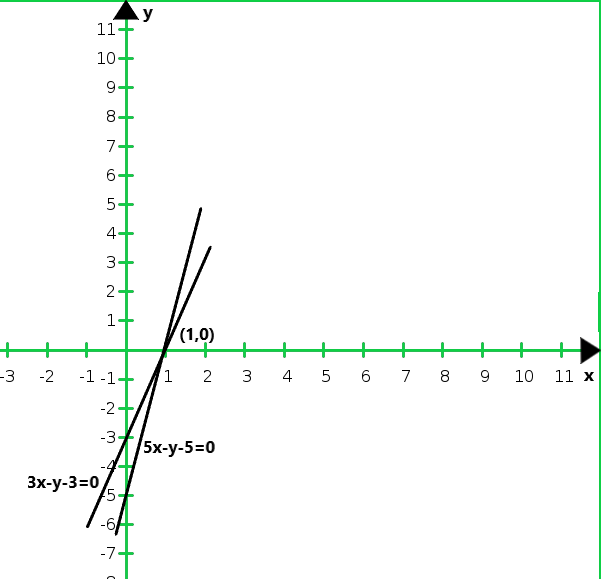It can be observed that the required triangle is ABC

Hence, the coordinates of its vertices A (1, 0) B(0, – 3) and C(0, -5)

Now, AB = 3.2, BC = 5.1

s = (a + b + c) /2 = (3.2 + 2 + 5.1)/2 = 5.2

Area of triangle ABC = √s(s – a)(s – b)(s – c)

= √5.2(5.2 – 3.2)(5.2 – 2)(5.2 – 5.1)

= √3.328

= 1.8 sq.unit

### Question 32. Form the pair of linear equation in the following problems, and find their solution graphically:

(i) 10 students of class X took part in the mathematics quiz. If the number of girls is 4 more than the number of boys. Find the number of boys and girls who took part in the quiz.

(ii) 5 pencils and 7 pens together cost Rs.50, whereas 7 pencils and 5 pens together cost Rs.46.

Find the cost of one pencil and one pen.

(iii) Champa went to a sale to purchase some pants and skirts. When her friends asked her how many of each kind she had bought, she answered, “the number of skirts is two less than twice the number of pants purchase”. Also, ” the number of skirts is four less than four times the number of pants purchased.” Help her friends to find how many pants and skirts Champa bought.

Solution:

(i) Let the number of girls and boys in the class be x and y respectively.

According to the question,

x + y = 10 and x – y = 4 are the given equations

Now, x + y = 10

x = 10 – y

So, the following table giving points on the line x + y = 10

Now, x – y = 4

x = 4 + y

So, the following table giving points on the line x – y = 4

So, the graph of the equations x + y = 10 and x – y = 4: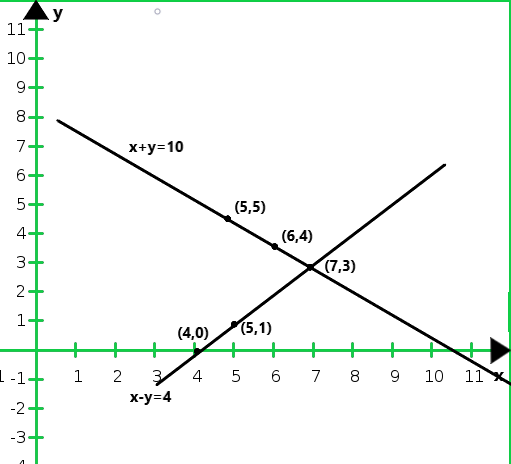From the graph we conclude that the two lines intersect each other at point (7, 3).

So, x = 7 and y = 3

Hence, the number of girls and boys in the class are 7 and 3 respectively.

(ii) Let the cost of one pencil and one pen Rs. x and Rs. y respectively.

According to the question, we get,

5x + 7y = 50

7x + 5y = 50

Now, 5x + 7y = 50

x = (50 – 7y)/5

So, the following table giving points on the line 5x + 7y = 50

Now, 7x + 5y = 46,

x = (46 – 5y)/7

So, the following table giving points on the line 7x + 5y = 46,

So, the graph of the equations 5x + 7y = 50 and 7x + 5y = 50: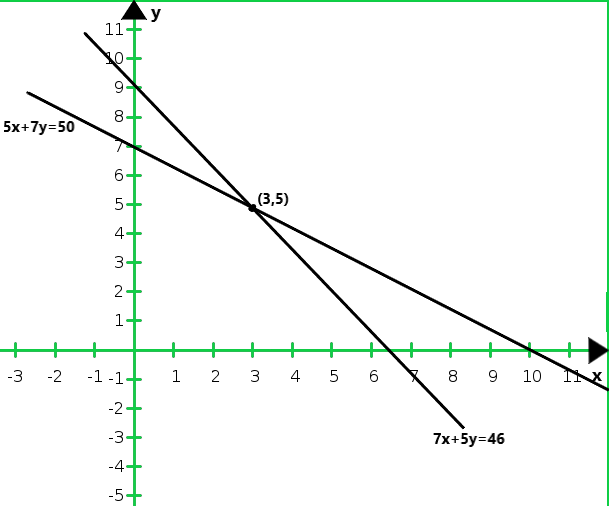From the graph we conclude that the two lines intersect each other at the point (3, 5)

So, x = 3 and y = 5

Hence, the cost of the pencil and the pen are 3 and 5 respectively.

(iii) Let us denote the number of pants by x and the number of skirts by y. Then the equations formed are :

y = 2x – 2    …..(i)

y = 4x – 2   ……(ii)

The graphs of the equations (i) and (ii) can be drawn by finding two solutions for each of the equations.

So, they are given in the following table.

Hence, the graphic representation is as follows: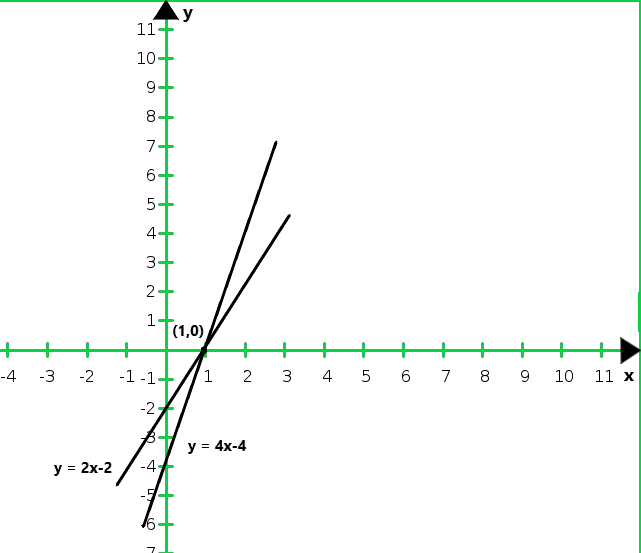The two lines intersect at the point (1, 0).

So, x = 1, y = 0 is the required solution of the pair of linear equations,

i.e., the number of parts she purchased is 1 and she did not buy any skirt.

### Shade the region between the lines and y-axis

(i) 3x – 4y = 7 and 5x + 2y = 3

(ii) 4x – y = 4 and 3x + 2y = 14

Solution :

(i) Given that, 3x – 4y = 7 and 5x + 2y = 3

Now, 3x – 4y = 7,

y = (3x – 7)/4

When x = 1, we get y = – 1

When x = – 3, we get y = – 4

So, the following table giving points on the line 3x – 4y = 7

Now, 5x + 2y = 3,

y = (3 – 5x)/2

When x = 1, we get y = – 1

When x = 3, we get y = – 6

So, the following table giving points on the line 5x + 2y = 3

So, the graph of the equations 3x – 4y = 7 and 5x + 2y = 3: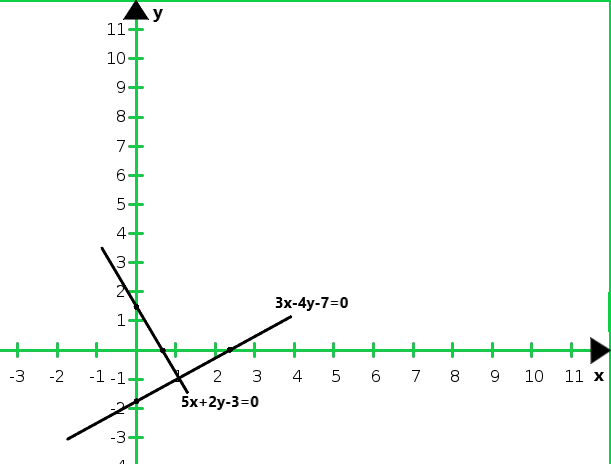From the graph we conclude that the two lines intersect at A(1,-1)

Hence, x = 1 and y = -1 is the solution of the given system of equations.

(ii) Given that, 4x – y = 4 and 3x + 2y = 14

Now, 4x – y = 4

y = 4x – 4

When x = 0, we get y = – 4

When x = -1, we get y = – 8

So, the following table giving points on the line 4x – y = 4

Now, 3x + 2y = 14,

y = (14 – 3x)/2

When x = 0, we get y = 7

When x = 4, we get y = 1

So, the following table giving points on the line 3x + 2y = 14

So, the graph of the equations 4x – y = 4 and 3x + 2y = 14: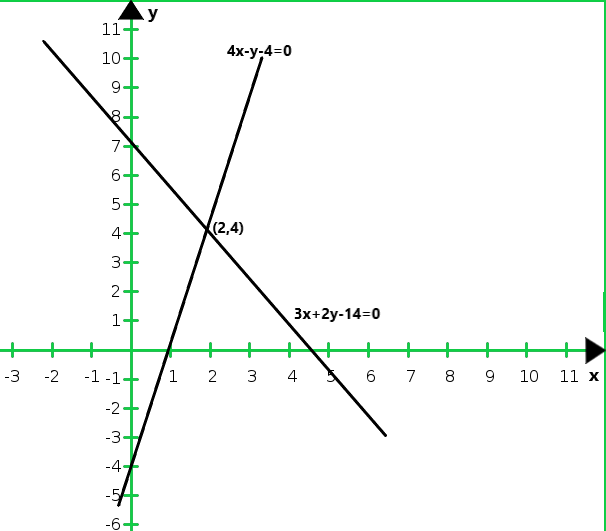From the graph we conclude that the two lines intersect at A (2, 4)

Hence, x = 2 and y = 4 is the solution of the given system of equations.

### x + 3y = 6 and 2x – 3y = 12

Solution:

Given that, x + 3y = 6 and 2x – 3y = 12

Now, x + 3y = 6,

y = (6 – x)/3

When x = 0, we get y = 2

When x = 3, we get y = 1

So, the following table giving points on the line x + 3y = 6

Now, 2x – 3y = 12,

y = (2x – 12)/3

When x = 0, we get y = – 4

When x = 6, we get y = 0

So, the following table giving points on the line 2x – 3y = 12

So, the graph of the equations x + 3y = 6 and 2x – 3y = 12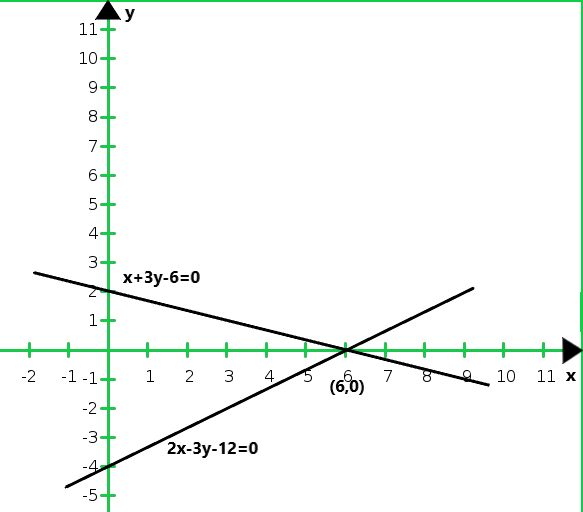From the graph we conclude that the two lines meet the y-axis at B(0, 2) and C(0, -4) respectively.

Hence, the required coordinates are (0, 2) and (0, -4)

### Question 35. Given the linear equation 2x + 3y – 8 = 0, write another in two variables in two variables such that the geometrical representation of the pair so formed is (i) intersecting lines (ii) parallel lines (iii) coincident lines.

Solution:

(i) For the two lines a1x + b1x + c1 = 0 and a2x + b2x + c2 = 0 to be intersecting.

We must have, a1/a2 ≠ b1/b2

So the other linear equation can be 5x + 6y – 16 = 0

a1/a2 = 2/5

b1/b1 = 3/6 = 1/2

c1/c2 = -8/-16 = 1/2

(ii) For the two lines a1x + b1x + c1 = 0 and a2x + b2x + c2 = 0 to be parallel

We must have, a1/a2 = b1/b2 ≠ c1/c2

So, the other linear equation can be 6x + 9y + 24 = 0

a1/a2 = 2/6 = 1/3

b1/b2 = 3/9 = 1/3

c1/c2 = -8/-24 = 1/3

(iii) For the two lines a1x + b1x + c1 = 0  and a2x + b2x + c2 = 0 to be coincident

We must have, a1/a2 = b1/b2 = c1/c2

So, the other linear equation can be 6x + 9y + 24 = 0

a1/a2 = 2/8 = 1/4

b1/b2 = 3/12 = 1/4

c1/c2 = -8/-32 = 1/4

Whether you're preparing for your first job interview or aiming to upskill in this ever-evolving tech landscape, GeeksforGeeks Courses are your key to success. We provide top-quality content at affordable prices, all geared towards accelerating your growth in a time-bound manner. Join the millions we've already empowered, and we're here to do the same for you. Don't miss out - check it out now!

Previous
Next